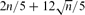Hostname: page-component-f7d5f74f5-4fqgl Total loading time: 0 Render date: 2023-10-02T05:53:28.056Z Has data issue: false Feature Flags: { "corePageComponentGetUserInfoFromSharedSession": true, "coreDisableEcommerce": false, "coreDisableSocialShare": false, "coreDisableEcommerceForArticlePurchase": false, "coreDisableEcommerceForBookPurchase": false, "coreDisableEcommerceForElementPurchase": false, "coreUseNewShare": true, "useRatesEcommerce": true } hasContentIssue false

# Triangles in Regular Graphs with Density Below One Half

Published online by Cambridge University Press:  01 May 2009

## Abstract

Let k3reg(n, d) be the minimum number of triangles in d-regular graphs with n vertices. We find the exact value of k3reg(n, d) for d betweenand n/2. In addition, we identify the structure of the extremal graphs.

Type
Paper
Information

## Access options

Get access to the full version of this content by using one of the access options below. (Log in options will check for institutional or personal access. Content may require purchase if you do not have access.)

## References

Ahlswede, R. and Katona, G. O. H. (1978) Graphs with maximal number of adjacent pairs of edges. Acta Math. Acad. Sci. Hungar. 32 97120.CrossRefGoogle Scholar
Andrásfai, B., Erdős, P. and Sós, V. T. (1974) On the connection between chromatic number, maximal clique and minimal degree of a graph. Discrete Math. 8 205218.CrossRefGoogle Scholar
Das, K. (2004) Maximizing the sum of the squares of the degrees of a graph. Discrete Math. 285 5766.CrossRefGoogle Scholar
de Caen, D. (1998) An upper bound on the sum of squares of degrees in a graph. Discrete Math. 185 245248.CrossRefGoogle Scholar
Lo, A. Cliques in graphs with bounded minimum degree. In preparation.Google Scholar
Nikiforov, V. (2007) The sum of the squares of degrees: Sharp asymptotics. Discrete Math. 307 31873193.CrossRefGoogle Scholar
Olpp, D. (1996) A conjecture of Goodman and the multiplicities of graphs. Austral. J. Combin. 14 267282.Google Scholar
Székely, L. A., Clark, L. H. and Entriger, R. C. (1992) An inequality for degree sequences. Discrete Math. 103 293300.CrossRefGoogle Scholar